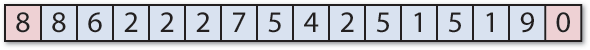## Tweetmaze

This uLisp program solves a logic maze puzzle consisting of a string of decimal digits. It's called a Tweetmaze because it's short enough to send in a tweet. For example, here's a 16-digit Tweetmaze: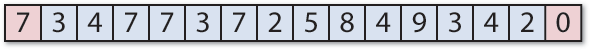You start from the leftmost digit, and the aim is to find a route to the last digit on the right in the shortest number of jumps. The number on each square tells you how far you can jump from that square, left or right, to the next square. So, for example, from the 7 on the starting square you can jump to the first 2 to its right, and then from that you can jump two squares to its left or right, and so on.

The program is an example of a problem that Lisp is suited to solving. With the addition of some hardware and control routines this program could form the basis for a maze-solving robot.

This revised version of the program will run on any of the platforms, including the Arduino Uno.

### The program

Here's the program to solve a Tweetmaze:

```(defun solve (maze)
(let ((go (1- (length maze)))
(queue '((0))))
(loop
(if (= (caar queue) go)
(return (reverse (car queue)))
(let* ((top (pop queue))
(pos (first top))
(rt (+ pos (nth pos maze)))
(lt (- pos (nth pos maze))))
(when (>= lt 0) (setq queue (append queue (list (cons lt top)))))
(when (<= rt go) (setq queue (append queue (list (cons rt top))))))))))```

The routine solve takes two parameters:

• maze, the maze to be solved, as a list of numbers.
• queue, the queue of paths taken so far, with the shortest path at the front.

It returns the solution, as a list of positions. Initially solve is called with queue set to the starting position, '((0)).

If the last cell visited by the shortest path is the goal, the routine returns the path. Otherwise it pops the shortest path from the front of the queue, and adds the two new longer paths, corresponding to going left and right from the current position, to the end of the queue.

Finally it calls solve again with the updated queue.

### First Tweetmaze

Here's an example of it solving the Tweetmaze shown above:

```> (solve '(7 3 4 7 7 3 7 2 5 8 4 9 3 4 2 0))
(0 7 5 8 3 10 14 12 15)```

Here's a graphical representation of this solution: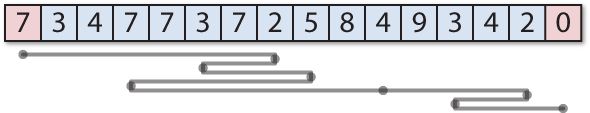### More Tweetmazes

Here are three more difficult Tweetmazes to solve. Try solving them by hand before you use the program to solve them.

#### Tweetmaze 2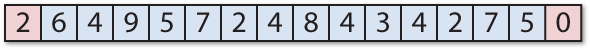#### Tweetmaze 3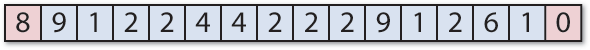#### Tweetmaze 4